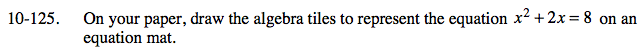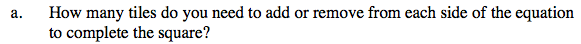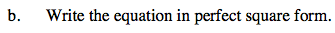### Home > AC > Chapter 10 > Lesson 10.4.1 > Problem10-125

10-125.Draw the equation mat with the algebra tiles.
In order to complete the square you will need to make the equation x2 + 2x + 1 on one of the sides.
What did you do to make this equation?x2 + 2x + 1 = 8 + 1
(x + 1)(x + 1) = 9
(x + 1)2 = 9